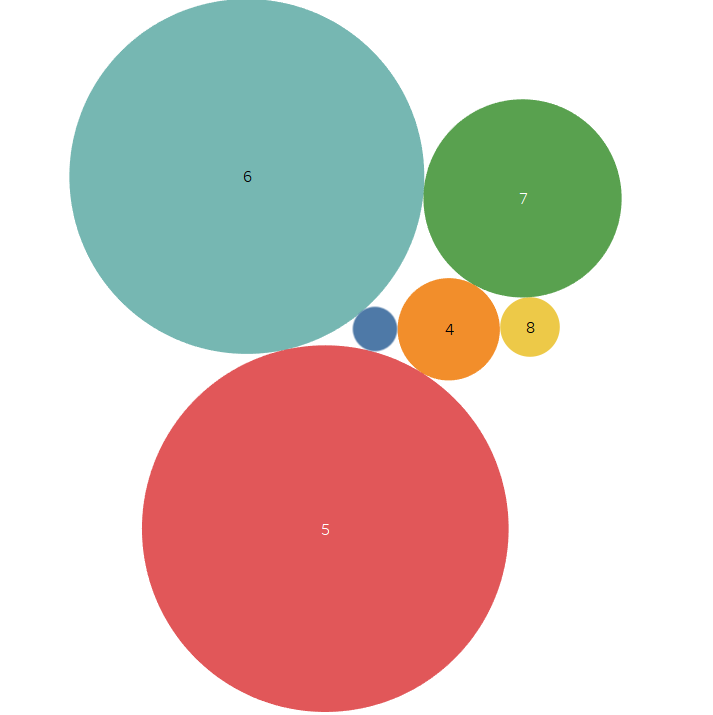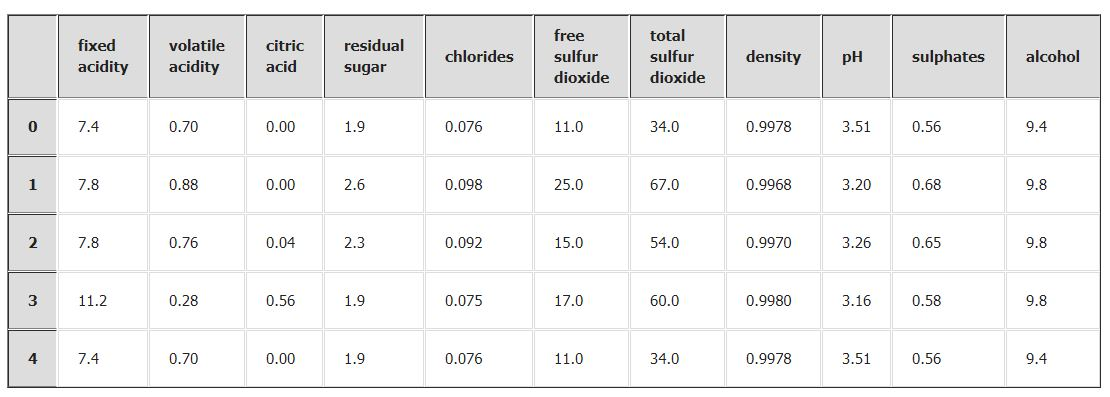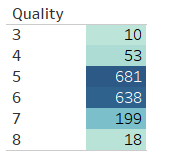# Imbalanced-Data Set for Classification

Machine Learning algorithms unsatisfied problem with classifiers when faced with imbalanced datasets. Let’s take an example of the Red-wine problem.The Data we have is as:Here we have a data set in which we have different six categories, but not balanced categories.So, in the case available the samples are not equally balanced so the accuracy will now meet up to expectation. Lets try with some Machine learning models:

Import Libraries,

```import pandas as pd
from sklearn.model_selection import train_test_split
from sklearn.linear_model import LinearRegression
from sklearn.cross_validation import cross_val_score
from sklearn.cross_validation import KFold,train_test_split,cross_val_score
from sklearn.linear_model import LogisticRegression```

```df= pd.read_csv('winequality-red.csv')
X=df.iloc[:,0:-1]
Y=df.iloc[:,-1:]```

Now, Split the data and implement Logistic regression,

`seed=23 x_train,x_test,y_train,y_test=train_test_split(X,Y,test_size=.3,random_state=seed) model=LogisticRegression(C=1.5,max_iter=300) model.fit(x_train,y_train) predict_train=model.predict(x_train) predict_test=model.predict(x_test)`
` `

Now Check the Accuracy of the classifier,

```import numpy as np
from sklearn.metrics import accuracy_score
acc_train=accuracy_score(predict_train,np.array(y_train))

acc_test=accuracy_score(predict_test,np.array(y_test))
acc_test.mean()
print('Train Acc',acc_train.mean())
print('Test Acc',acc_test.mean())```

Output:

```Train Acc: 0.573726541555
Train Acc: 0.602083333333```

It’s not Good, so let’s try some other classifier like Decision Tree:

```from sklearn.tree import DecisionTreeClassifier
clf_dt=DecisionTreeClassifier(random_state=5)
clf_dt.fit(x_train,y_train)
#results=cross_val_score(clf_dt,x_train,y_train)
y_bag=clf_dt.predict(x_test)
score=accuracy_score(y_bag,y_test)
print(score)```

Output:

```0.6
```

This signifies that model is underfitting and we are not able to fit anyway as there is an issue in the proportion of data so we have to use Ensemble methods.

What is Ensemble Methods,

• Ensemble methods enable combining different models or classifiers into the single model to create a robust generalized model.
• Ensemble methods combine different models to cancel out their individual weakness, which often result in the stable and well-performing model that are very attractive for industrial applications and as well as machine learning competitions.

Bootstrapping is a sampling technique with replacement. This ends up leaving some data unselected (on average 63% are sampled), while the remaining 37% of the training instances that are not sampled are called out-of-bag instances. Since the predictor never sees the out of bag instances during training, it can be evaluated in these instances without the need for a separate validation set or cross-validation.

Creating multiple models on the same set of observations will give approximately the same results. Therefore, the whole set is broken down into smaller sets, (with replacement). This process of breaking down into multiple sets is bootstrapping.

Different Ensemble methods are:

Combine multiple models of similar types,

• Bagging (Bootstrap aggregations)
• Various algorithms which use bagging technique are Bagging estimator, Random Forest, Extra trees.
• Boosting
• AdaBoost GBM, XGBoost, etc are some of the algorithms which use boosting technique.

Combining multiple models of various types,

• Vote classification
• Blending or stacking

### Bagging

• Bagging is a model aggregation techniques to reduce model variance.
• More complex task and dataset’s high dimensionality easily lead to overfitting in the single decision tree and there is where bagging plays out its strength.
• Bagging is an effective approach to reduce variance but ineffective in case of bias.
• In this training data is split into multiple datasets with a replacement called bootstrap.
• The bootstrap sample size will be the same of training data size.
• Independent model on bootstrap sample built an average for the regression case or majority vote or mode for the classification is used to create final model.
• Let suppose n be the number of bootstrap samples created out of original training set. For i=1 to N train a base machine learning model ci.
• C_final=aggregate max of y å I (C_i=y)

Bagging Code,

```from sklearn.ensemble import BaggingClassifier
num_trees=121
model_bag=BaggingClassifier(base_estimator=clf_dt,n_estimators=num_trees,random_state=5,
bootstrap_features=True,n_jobs=2,max_samples=1.0)
model_bag.fit(x_train,y_train)

y_bag=model_bag.predict(x_test)
score=accuracy_score(y_bag,y_test)
print(score)```
`0.7`

Random Forest,

```from sklearn.ensemble import RandomForestClassifier
clf_RF=RandomForestClassifier(n_estimators=num_trees)
clf_RF.fit(x_train,y_train)
y_ran=clf_RF.predict(x_test)
y_bag=clf_RF.predict(x_test)
score=accuracy_score(y_bag,y_test)
print(score)```
`0.708333333333`

Extra Tree,

```from sklearn.ensemble import ExtraTreesClassifier
clf_ET=ExtraTreesClassifier(n_estimators=num_trees)
clf_ET.fit(x_train,y_train)
y_ET=clf_ET.predict(x_test)
y_bag=clf_ET.predict(x_test)
score=accuracy_score(y_bag,y_test)
print(score)```
`0.71875`

#### Boosting

• The objective behind boosting is that rather than an independent individual hypothesis combining hypothesis in sequential order increase the accuracy.
• Essentially boosting algorithms convert weak learner into strong.
• Boosting is well designed to address bias problems.
• There are four step used for boosting
• Draw a random subset of training samples d1 without replacement from the training set D  to train a weak learner c1.
• Draw second random training set without replacement and add 50% of the sample that was previously misclassified to train a weak learner c2.
• Find a training sample d3 on which c1 and c2 disagree to train a third weak learner.
• Combine the weak learners c1,c2,c3 by majority voting.

```from sklearn.ensemble import AdaBoostClassifier
score=accuracy_score(y_bag,y_test)
print(score)```
`0.727083333333`

```from sklearn.ensemble import GradientBoostingClassifier
`0.695833333333`